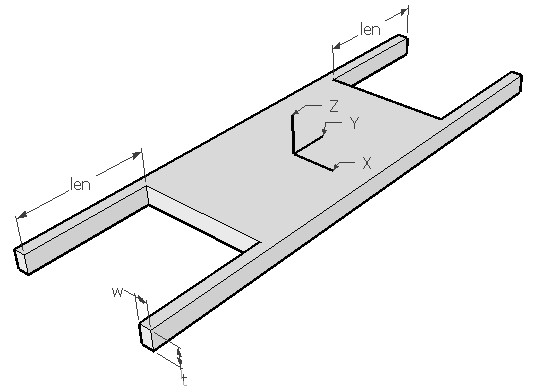GUIDBEAM,len,w,t,ymod,sel
Design of a guided beam hammock suspension

len         length of the beam in µm
w           width of the beam in µm
t            thickness of the beam in µm
ymod    Young's modulus of the material of the beam in GPa
sel         number denoting the selected result.
Use 1 for spring constant in X axis and 2 for spring constant in Y axis and 3 for spring constant in Z axisNotes

A hammock suspension, sometimes called as fixed-fixed flexure shown above is formed of four identical guided beams joined at the corner of a central mass. Guided beam is a common type of beam used in MEMS which has one end fixed and the other end is guided. For analysis, each guided beam can be considered as two cantilevers in series.

In this analysis all four beams are considered in calculating the effective spring constant of the suspension. If you wish to find the spring constant for just one beam, divide the result by 4 since the four beams are in parallel.

The suspension allows lateral movement in the X axis and vertical movement in Z axis. It is often used for comb drives, MEMS switches and MEMS varactor. It also has an out-of-plane movement perpendicular to the plane of the mass in Z direction. The stiffness in these two directions can be varied by changing the width to thickness ratio of the beam. This suspension is very stiff in the axial Y direction, but introduces a non-linear factor to it. The stiffness of this suspension in all the three axis directions can be found out using this design interface.

The plot shows lateral to vertical stiffness ratio (stiffness in X over Z) over the ratio of width to thickness (w / t) of the beam when the length is held constant. Increase the thickness to improve the sensitivity to lateral movement.

Assumptions

-The beam has uniform rectangular cross section.
-The beam has small deflection and has small angle or rotation
-No axial loading and no shear deformation.
-Residual stress is not considered.  The beam will buckle if residual stress is more than the critical stress.
-Only bending effects are considered. Axial deformations are assumed to be small compared to bending effects.